## Using a Raspberry Pi Pico to record how long it takes for a battery to drain

In this tutorial we’ll demonstrate how a Raspberry Pi Pico can be used along with some simple electronic components and Python code to record the voltage of a AA battery. This project can be used to gather data about how long it takes a AA battery to drain. As the battery level drains the voltage output by it is reduced. We can read the batteries voltage by connecting it to an ADC pin on the Raspberry Pi Pico.

## Wire up the Pico

To setup the Pico for this project we first place it on one edge of our breadboard with the Micro USB connector facing out. We will need to power the Pico using the USB cable so it is helpful the have the connector easy to access for when we run our tests.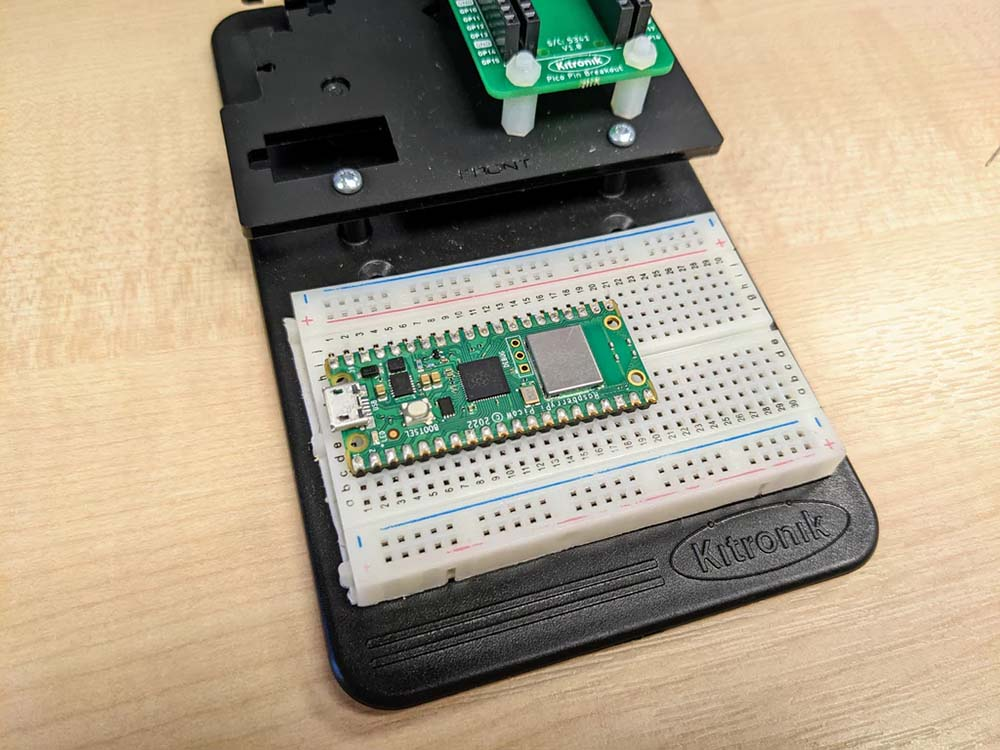Then add the 10k Ω resistor. We want it connecting GPIO 26 on the Pico to the power line on the breadboard. We also want to connect one of the ground pins on the Pico to the ground line on the breadboard. This will link up the ground on the Pico to the battery's negative pin.Next add the four 10 Ω resistors to the power line on the breadboard. Then using our four jumper wires we'll connect the 10 Ω resistors to the ground line on the other side of the breadboard. We use only 10 Ω resistors as we want the load on the battery to be as high as possible. We therefore want to use high power resistors to reduce the chance of them breaking while under high load. Even with high power resistors they will still get hot and shouldn't be touched while the battery is connected, or after the battery has been connected for an extended period of time.

Using a low resistance means that lots of current will be able to flow from the battery, and so the battery will drain quicker. Using four 10 Ω resistors in parallel like this gives the circuit a 2.5 Ω resistance, putting an even greater drain on the battery.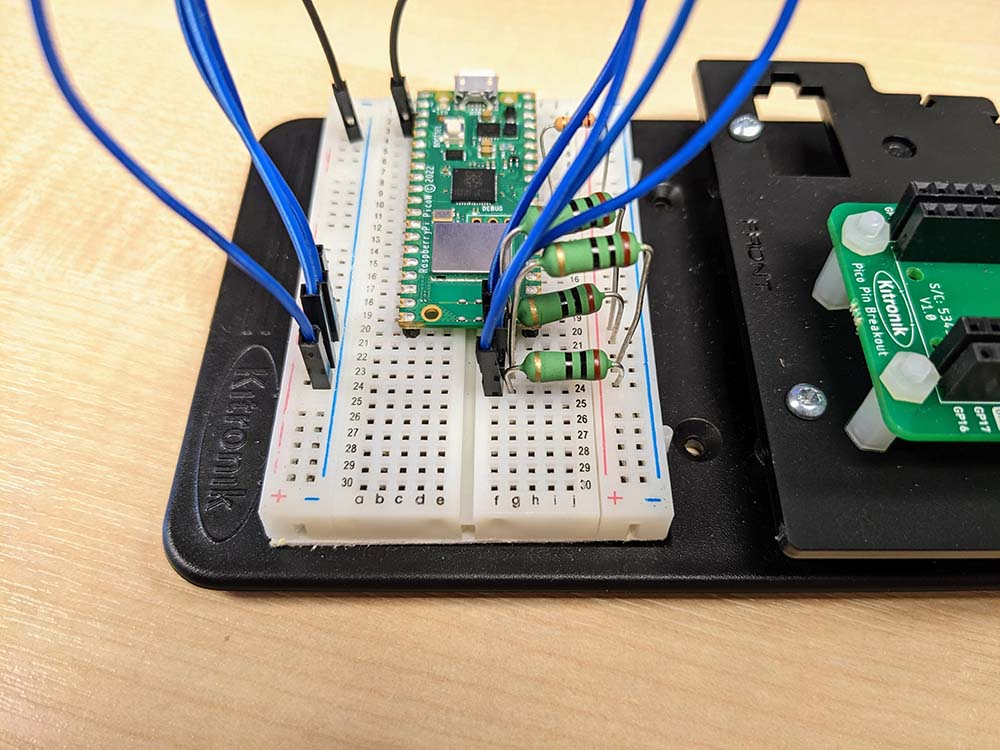Finally, we’ll add the battery cage with the AA battery to the breadboard. Here we add the battery cage negative wire to the negative line on the breadboard and the power wire to the power line on the breadboard.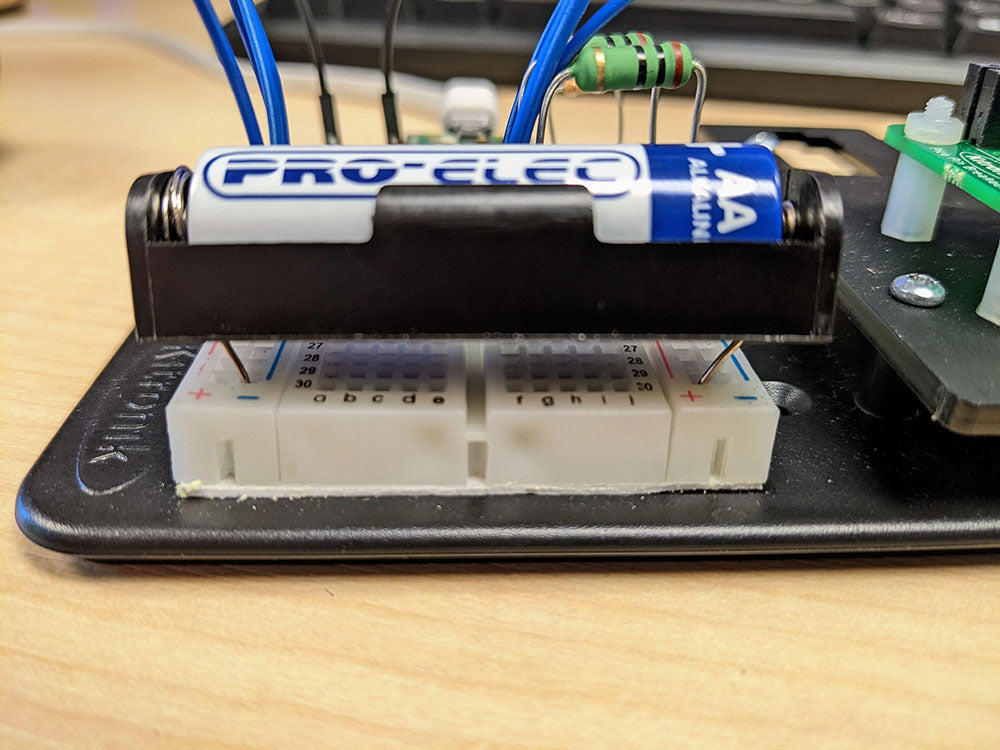In this circuit we are using the Pico to measure the discharge of the battery. The Pico is not causing the discharge on the battery. It is simply going to be measuring the output voltage of the battery as regular intervals. The discharge on the battery is actually caused by four resistors we have added in parrallel to the circuit.

Below is the complete circuit diagram for our Raspberry Pi Pico Battery Voltmeter.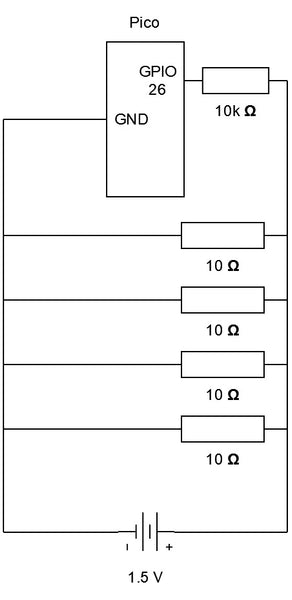## Code the Pico

### Imports

To code the voltmeter we’ll use two imports. As our voltage sensor we’ll use an ADC pin on the Pico. To access the Pico's ADC pins we'll use an ADC object from the machine library. Then while monitoring the voltage of the battery we need to delay the loop so that the voltage readings are taken at regular intervals. To do this we can use the sleep function from the time library inside of our voltage reading loop.

``````from machine import ADC
from time import sleep
``````

### Variable Setup

Our code will need some global variables to keep track of our voltage readings. The analogIn variable will store our ADC object which we set to use GPIO pin 26 as this is ADC pin 0. To read our voltage we use ADC pin as these allow for analogue data (our battery’s voltage) to converted to a digital value by taking a reading of the voltage on the pin.

The minutes and seconds variables will store how long we have been reading the battery’s voltage and allow us to save this alongside our voltage data to see how long it takes to drain the battery.

``````analogIn = ADC(26)
minutes = 0
seconds = 0
``````

### Helper Function

To separate our code we’ll create a helper function called writeLine that will write our voltage readings to a file. The writeLine function should take text as an input parameter. We need to open a file in append mode, “a”, to add text to the end of the file without erasing it's contents. We can call our file “voltmeter.txt” and store it in the variable 'file'.

Next use 'file' to write our input text into the file and concatenate a new line at the end of the text. Adding the new line ensures that each voltage reading is on its own line in our file. Finally, close 'file' to save our newly written text. Our writeLine function can optionally output the text to the console using print.

``````def writeLine(text):
file = open("voltmeter.txt", "a")
file.write(text + "\n")
file.close()    print(text)``````

### Voltage Sensor Loop

To record our battery’s voltage we'll check it at a regular interval of 1 second. To do this we’ll use an infinite loop, done by setting a while loop's condition to True. Inside the loop we want to read the current value at our ADC pin and store it in the sensorValue variable. To convert this value into our battery's voltage we’ll need to do some simple maths.

The ADC pins convert the analogue input into an unsigned 16 bit integer meaning the maximum reading from the input will be 65535 with a minimum reading of 0. The Raspberry Pi Pico GPIO pins allow for voltages from 0.0 V to 3.3 V. With this we can scale our ADC input by multiplying the sensorValue by 3.3 V divided by 65535 and store this in the voltage variable. Now we can store the voltage reading alongside the current minute and second using our writeLine helper function.

Next we need to increase the number of seconds our voltmeter has been running by 1. When the seconds reaches 60 we need to increase the number of minutes running by 1 and reset the seconds back to 0. Finally, we’ll delay the next iteration of the loop by 1 second using the sleep function, before the whole voltage reading loop runs again.

``````while True:
voltage = sensorValue * (3.3 / 65535)
writeLine(str(minutes) + "," + str(seconds) + "," + str(voltage))
seconds += 1
if (seconds == 60):
seconds = 0
minutes += 1
sleep(1)
``````

## Voltmeter Data Plot

Here is an example of the voltage data gathered for two 1.5 V AA batteries using the Raspberry Pi Pico voltmeter project. I took 2 hours of voltage readings for each battery. The first battery managed to still output just below 1.0 V after the 2 hours. The second battery however was only outputting around 0.5 V after the 2 hours.

For the first minute I had the voltmeter running on the battery without the load resistors connected. From this we see the highest voltage both batteries give off at about 1.5 V. Then I added the load resistors where there is a sharp voltage drop for both batteries near the start. This happens because of the current being pulled by the four 10 Ω resistors in parallel. Once there is work being done in the circuit the actual voltage of the battery is lower than its open circuit voltage (OCV), which is how batteries are rated.

When the load resistors are added, the voltage then begins to drop at a steady rate as the resistors use the energy in the circuit. There are several points in the graph we see the voltage fluctuates suddenly. This was likely caused by the jumper wires being loose and interfering with the connection.

For most of the time the first battery was being recorded, the voltage was generally quite consistent and only decreases slightly over the span of a couple hours. The steepest part of the graph for the first battery can be seen in the first 15 minutes of the voltmeter running. Here the battery drains the fastest, before it slowly flattens out over the rest of the time the voltage was being recorded.

For the second battery however, it's voltage was on a gentle decline for the entire 2 hours it was being recorded. The rate at which the battery drains is very consistent, but again has the fastest drain happens within the first 15 minutes of connecting the battery to our voltmeter circuit.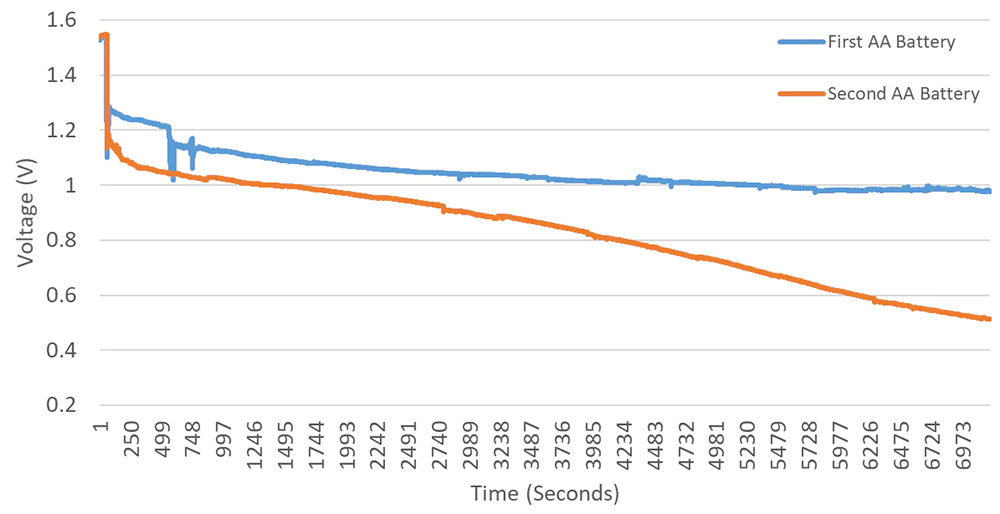The voltage read from the first battery after the 2 hours is double the voltage read from the second battery. This definitely does suggest that the first battery lasts longer than the second. It is difficult to know how much longer though, without running the voltmeter until both batteries are fully drained.

It is also important to consider that different types of batteries drain in different ways as the first battery is an alkaline battery, and the second a zinc-chloride battery. Try different types of battery - see if rechargeable batteries are better than alkaline batteries.

The alkaline battery tries to maintain a reasonable voltage while it still has charge. Once the charge stored in the battery gets too low it can no longer supply the same level of voltage. At this point the voltage drops suddenly and the battery runs out of charge. We don't get to see this on our graph as the voltmeter had only been on for 2 hours, but if ran for longer this would be the case.

We can also see in the graph that once the load resistors had been added, the second battery was already supplying a lower voltage than the first battery. Within the first few minutes the first battery was supplying about 1.25 V and the second battery was already supplying below 1.15 V.

## Conclusion

Now you have a simple Raspberry Pi Pico project that can be used as a voltmeter on a battery.

The draining of the battery can be slowed down by using a higher amount of resistance to reduce the current flowing from the battery.

Note: This method for reading the voltage of a battery may not be 100% accurate and is intended to only be used for demonstration purposes.

## Full Code

``````from machine import ADC
from time import sleep

minutes = 0
seconds = 0

def writeLine(text):
file = open("voltmeter.txt", "a")
file.write(text + "\n")
file.close()
print(text)

while True: